# Homogeneous Linear Differential Equations

## Homogeneous Linear Differential Equations:

Consider an nth order homogeneous linear differential equations with constant coefficients,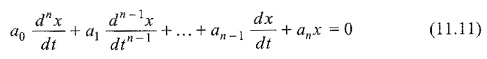where a0, a1 … an are real constants.

Now we shall find the solution of Eq. 11.11 of the form x = emt. By assuming that x = emt is a solution for certain m, we have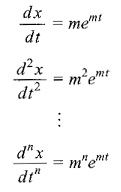Substituting in Eq. 11.11, we get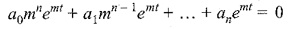or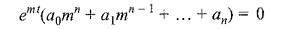where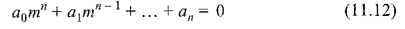This is called the auxiliary, or the characteristic equation of the given Homogeneous Linear Differential Equations. Three cases might occur in the auxiliary equation which are, subject to the roots of Eq. 11.12 being real and distinct, real and repeated, or complex.

#### Case 1 Distinct real roots:

If the roots of the Eq. 11.12, m1, m2 … mn are real and distinct, the general solution of Eq. I 1.11 is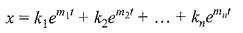where k1, k2 … kn are arbitrary constants.

k1, k2 … kn values can be determined by using initial conditions.

#### Case 2 Roots are real and repeated:

If the roots of Eq. 11.12 are the double real root m, and (n -2) distinct real roots.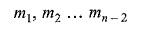then the linearly independent solutions of Eq. 11.11 are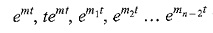And the general solution may be written as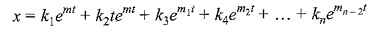Similarly, if Eq. 11.12 has a triple real root m, the general solution is (c1 + c2t + c3t2) emt.

#### Case 3 Roots are Complex Conjugate:

Consider the auxiliary equation which has the complex number a + jb as a non-repeated root. The corresponding part of the general solution is p1e(a+jb)t + p2e(a-jb)t, where p1 and p2 are arbitrary constants.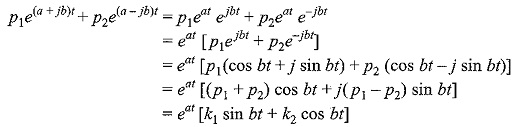where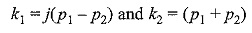are the new arbitrary constants.

If however, (a + jb) and (a — jb) are each n roots of the auxiliary equation, the corresponding general solution may be written as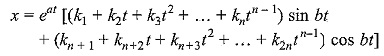Updated: December 29, 2019 — 12:14 pm